## A solid nonconducting sphere of radius R has a charge Q uniformly distributed throughout its volume. A Gaussian surface of radius r with r &

Question

A solid nonconducting sphere of radius R has a charge Q uniformly distributed throughout its volume. A Gaussian surface of radius r with r < R is used to calculate the magnitude of the electric field E at a distance r from the
center of the sphere. Which of the following equations results
from a correct application of Gauss’s law for this situation? And Why?

1. E (4 p r^2) = (Q r^3)/( epsilon sub0 * R3)
2. E(4pr^2)= (Q 3r^3)/ (epsilon sub0 * 4 p R)
3. E (4 p r^2) = (Q) / (epsilon sub0)

in progress 0
2 months 2021-08-22T11:07:45+00:00 1 Answers 3 views 0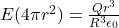or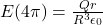Explanation:

We know that Gauss’s law states that the Flux enclosed by a Gaussian surface is given by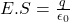Here , E is electric field and S is surface are and q is charge enclosed by the surface and e is electrical permeability of the medium.

Here the Gaussian is of radius r<R so area of surface is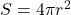Also, charge enclosed by the surface is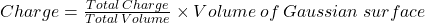therefore,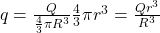Here Q is total charge,

Insert values in Gauss’s law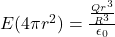Rearrange themon further solvingThis is the required form.